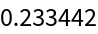Function Repository Resource:

# SphericalIncircle

Generate the incircle of a spherical triangle

Contributed by: Jan Mangaldan
 ResourceFunction["SphericalIncircle"][{p1,p2,p3}] returns a BSplineCurve representing the incircle of the spherical triangle with corner points p1,p2, and p3. ResourceFunction["SphericalIncircle"][{p1,p2,p3},property] gives the value of the specified property.

## Details

The points pi are taken to be unit vectors (i.e., on a unit sphere centered at the origin).
The following properties are supported:
 "Association" association containing the incircle, incenter and inradius "Center" center of the incircle (in Cartesian coordinates) "Circle" BSplineCurve representing the incircle "Radius" inradius (spherical distance)

## Examples

### Basic Examples (3)

Generate the vertices of a spherical triangle:

 In:=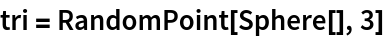Out=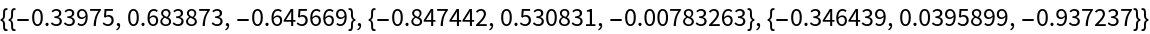Show the incircle as a BSplineCurve object:

 In:=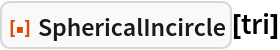Out=Visualize the spherical triangle and its incircle together:

 In:=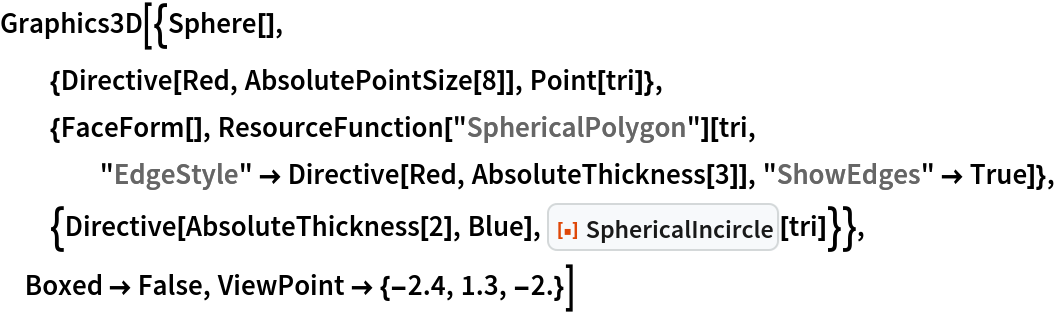Out=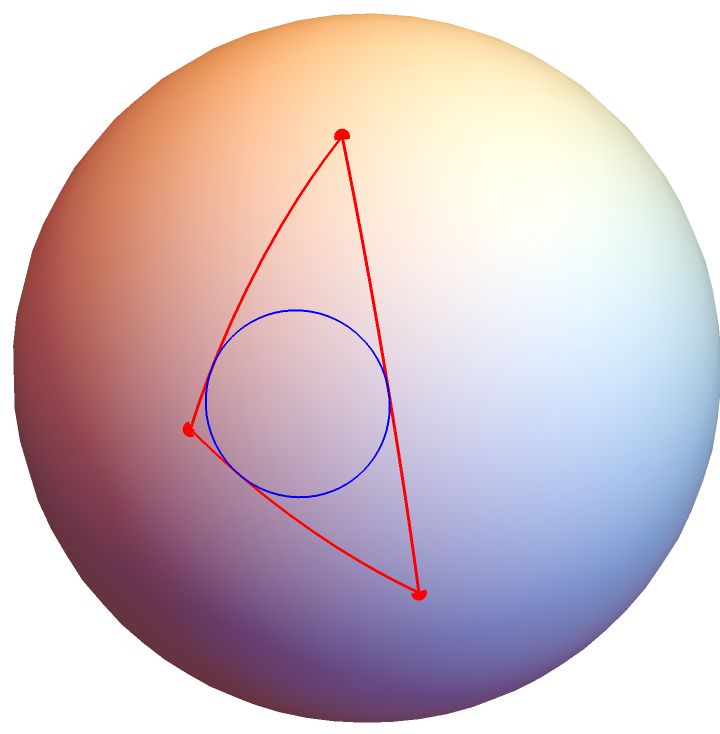### Scope (2)

Generate the vertices of a spherical triangle:

 In:=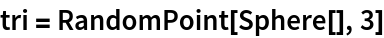Out=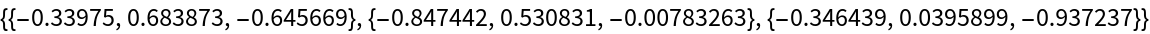In:=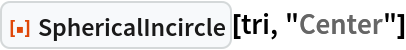Out=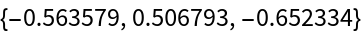In:=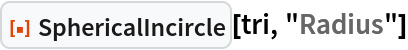Out=